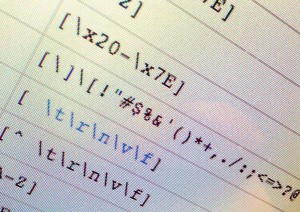Password constraints can be one of the most complicated applications we can perform using regular expressions, but fortunately, we have some experience to make our task easier.

#### Assume we would like our password to contain all of the following, but in no particular order:

1. At least one digit `[0-9]`
2. At least one lowercase character `[a-z]`
3. At least one uppercase character `[A-Z]`
4. At least one special character `[*.!@#\$%^&(){}[]:;<>,.?/~_+-=|\]`
5. At least 8 characters in length, but no more than 32.

To solve this, we turn to our friends, the look-ahead, which when combined with logical and in regex, allows us to achieve the desired result. We have a regular expression that enforces all of the above constraints, but allows us to satisfy them in any order, whether or not the digits, letters, or special characters come first, last, or anywhere:

### The pattern:

`^(?=.*[0-9])(?=.*[a-z])(?=.*[A-Z])(?=.*[*.!@\$%^&(){}[]:;<>,.?/~_+-=|\]).{8,32}\$`

#### Dissecting the pattern

```^ Match the beginning of the string (?=.*[0-9]) Require that at least one digit appear anywhere in the string (?=.*[a-z]) Require that at least one lowercase letter appear anywhere in the string (?=.*[A-Z]) Require that at least one uppercase letter appear anywhere in the string (?=.*[*.!@\$%^&(){}[]:;<>,.?/~_+-=|\]) Require that at least one special character appear anywhere in the string .{8,32} The password must be at least 8 characters long, but no more than 32 \$ Match the end of the string.```

## Enforcing several of many constraints

Let’s now consider the situation where we want to enforce 3 out of 4 of our character constraints on each password. We can still achieve this with regular expressions, but as you can see, things quickly get confusing.

### The enhanced pattern:

`^(?:(?:(?=.*[0-9])(?=.*[a-z])(?=.*[A-Z]))|(?:(?=.*[a-z])(?=.*[A-Z])(?=.*[*.!@\$%^&(){}[]:;<>,.?/~_+-=|\]))|(?:(?=.*[0-9])(?=.*[A-Z])(?=.*[*.!@\$%^&(){}[]:;<>,.?/~_+-=|\]))|(?:(?=.*[0-9])(?=.*[a-z])(?=.*[*.!@\$%^&(){}[]:;<>,.?/~_+-=|\]))).{8,32}\$`

Here we have used non-capturing groups `(?: ...)`, combined with alternation `|` to ensure that at least one set of three out of four constraints is met; however, this means a good deal of duplication without our pattern. Thus a more manageable solution would be to implement such a check using the higher programming language of your choice, or in this case, Java:

## Solution via a higher language

```/**
* Ensure that we have at least three out of four password criteria met.
* This would far more complicated to achieve using standard regular expressions.
*/
public boolean passwordValidates( String pass ) {
int count = 0;

if( 8 <= pass.length() && pass.length() <= 32  )
{
if( pass.matches(".*\\d.*") )
count ++;
if( pass.matches(".*[a-z].*") )
count ++;
if( pass.matches(".*[A-Z].*") )
count ++;
if( pass.matches(".*[*.!@#\$%^&(){}[]:";'<>,.?/~`_+-=|\\].*") )
count ++;
}

return count >= 3;
}```

1.Andrea Aime says:
1.Lincoln Baxter III says: